Скачать презентацию gravitational dirac bubbles stability and Mass splitting Shimon

a412e2eba5f4abe062c706a5f60c8122.ppt

• Количество слайдов: 38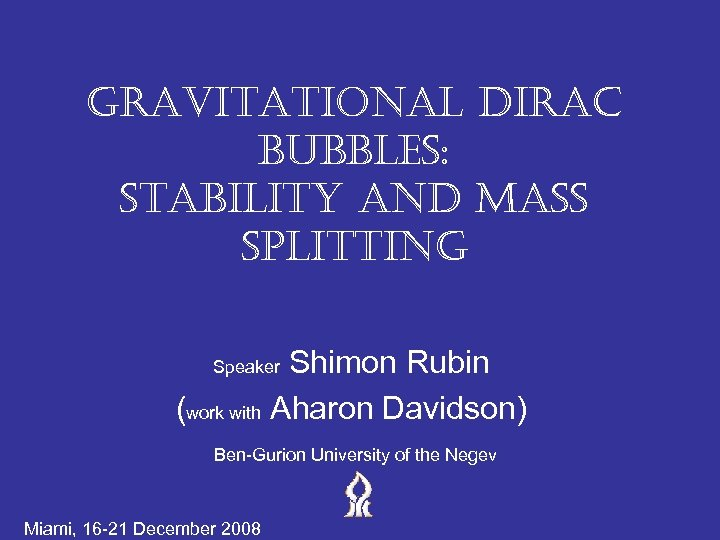gravitational dirac bubbles: stability and Mass splitting Shimon Rubin (work with Aharon Davidson) Speaker Ben-Gurion University of the Negev Miami, 16 -21 December 2008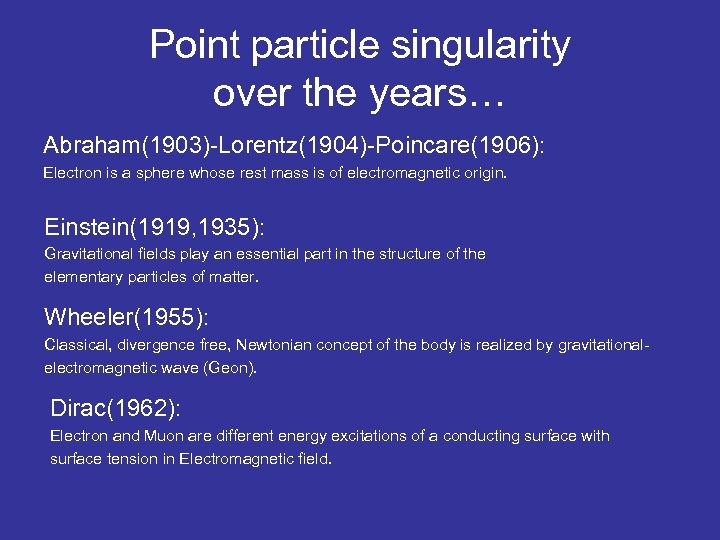Point particle singularity over the years… Abraham(1903)-Lorentz(1904)-Poincare(1906): Electron is a sphere whose rest mass is of electromagnetic origin. Einstein(1919, 1935): Gravitational fields play an essential part in the structure of the elementary particles of matter. Wheeler(1955): Classical, divergence free, Newtonian concept of the body is realized by gravitationalelectromagnetic wave (Geon). Dirac(1962): Electron and Muon are different energy excitations of a conducting surface with surface tension in Electromagnetic field.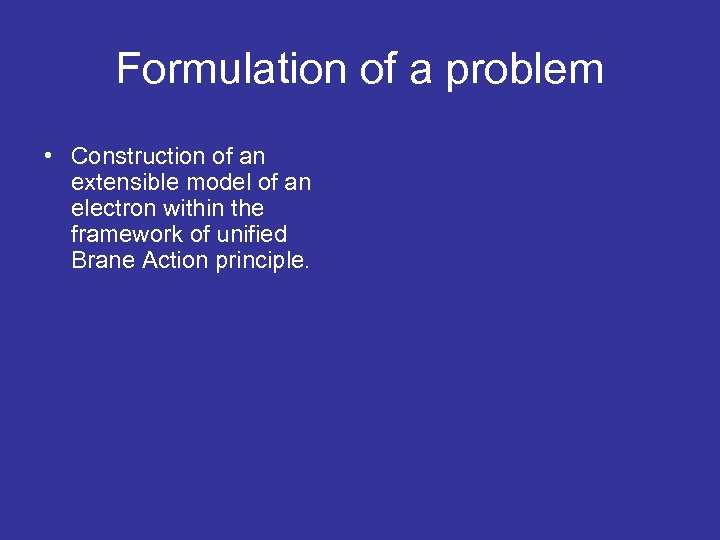Formulation of a problem • Construction of an extensible model of an electron within the framework of unified Brane Action principle.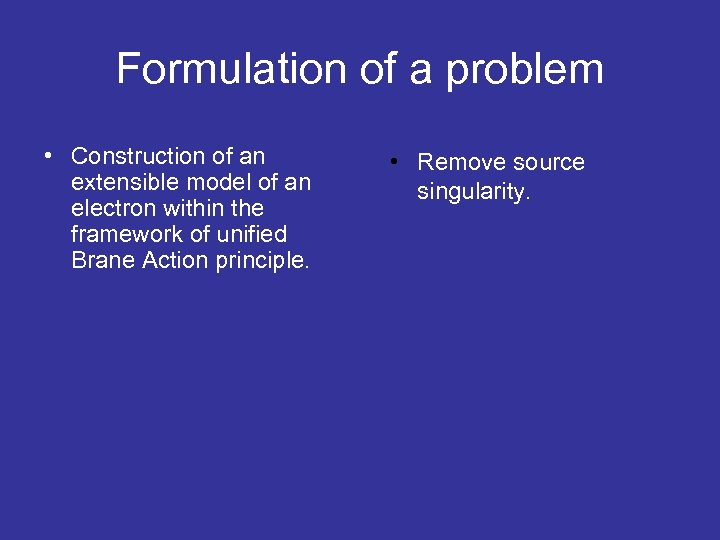Formulation of a problem • Construction of an extensible model of an electron within the framework of unified Brane Action principle. • Remove source singularity.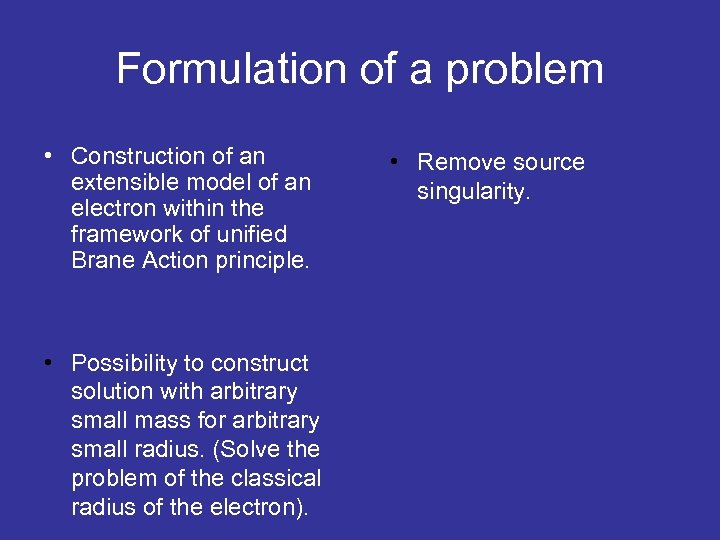Formulation of a problem • Construction of an extensible model of an electron within the framework of unified Brane Action principle. • Possibility to construct solution with arbitrary small mass for arbitrary small radius. (Solve the problem of the classical radius of the electron). • Remove source singularity.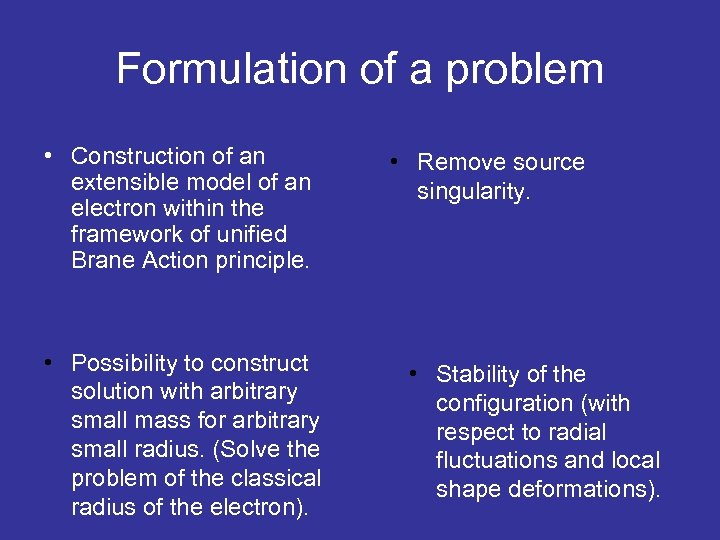Formulation of a problem • Construction of an extensible model of an electron within the framework of unified Brane Action principle. • Possibility to construct solution with arbitrary small mass for arbitrary small radius. (Solve the problem of the classical radius of the electron). • Remove source singularity. • Stability of the configuration (with respect to radial fluctuations and local shape deformations).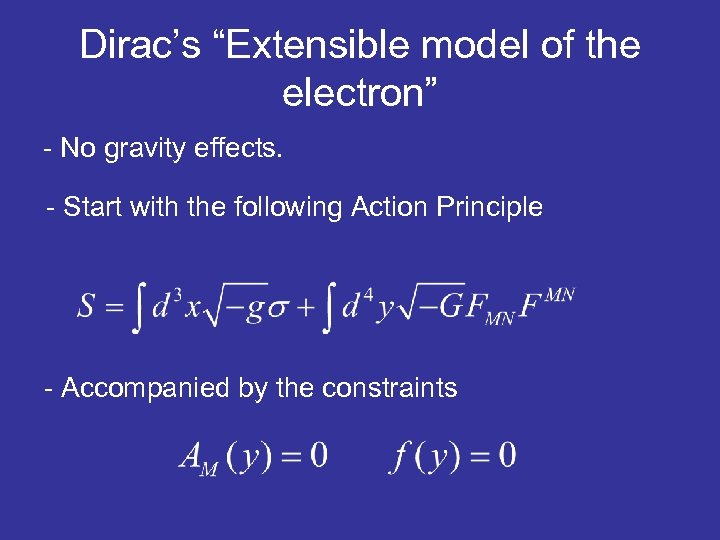Dirac’s “Extensible model of the electron” - No gravity effects. - Start with the following Action Principle - Accompanied by the constraints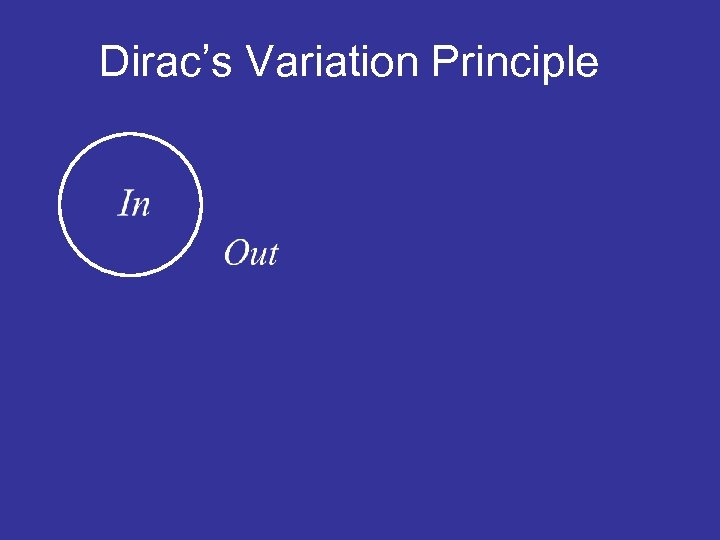Dirac’s Variation Principle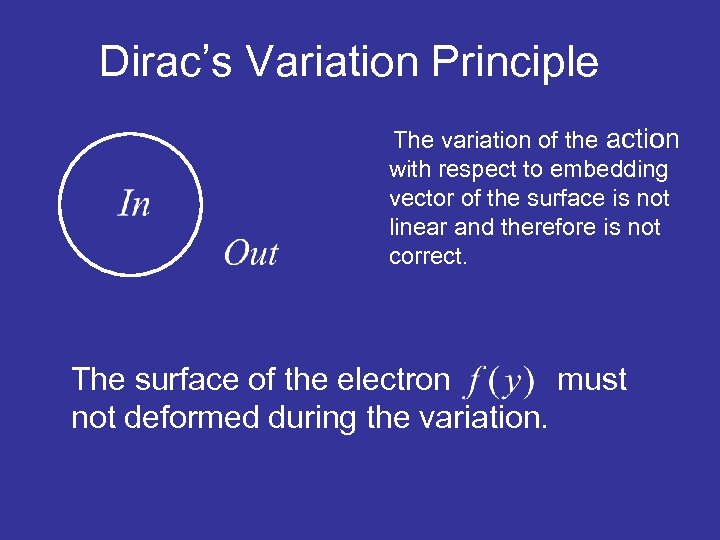Dirac’s Variation Principle The variation of the action with respect to embedding vector of the surface is not linear and therefore is not correct. The surface of the electron must not deformed during the variation.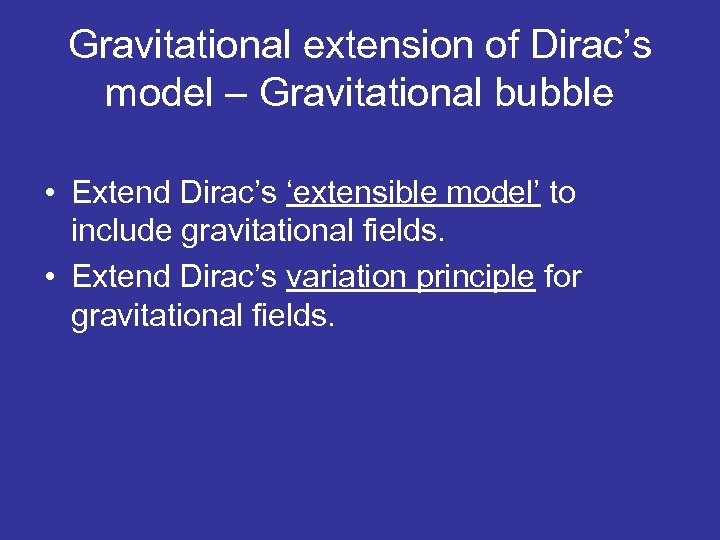Gravitational extension of Dirac’s model – Gravitational bubble • Extend Dirac’s ‘extensible model’ to include gravitational fields. • Extend Dirac’s variation principle for gravitational fields.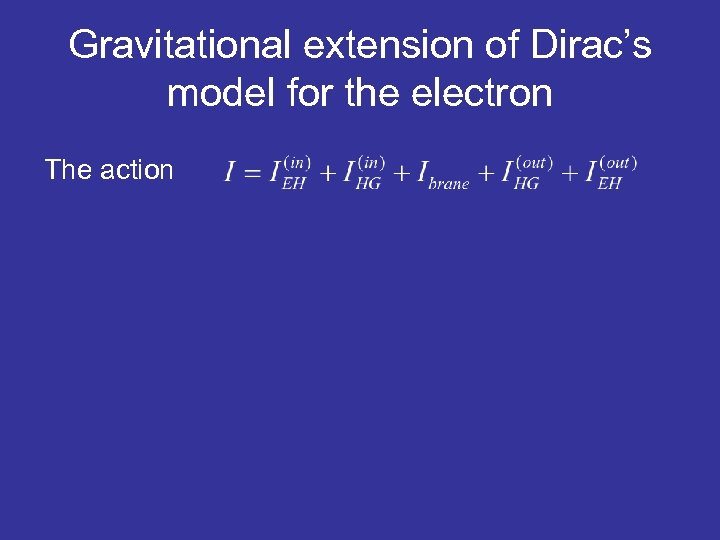Gravitational extension of Dirac’s model for the electron The action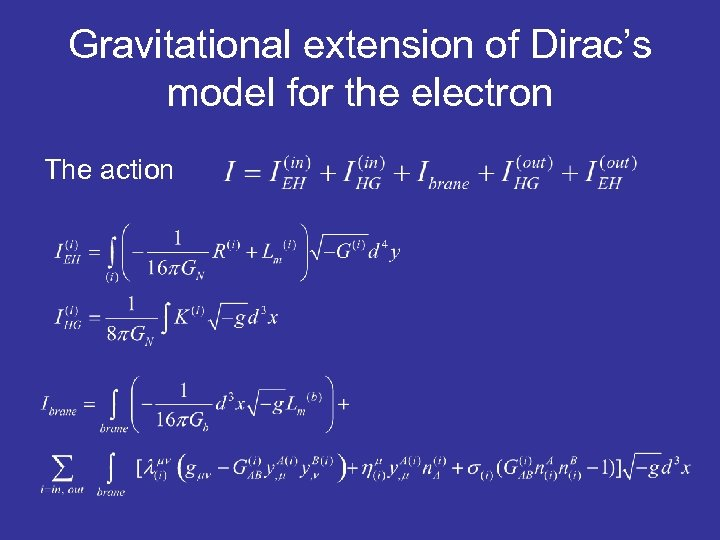Gravitational extension of Dirac’s model for the electron The action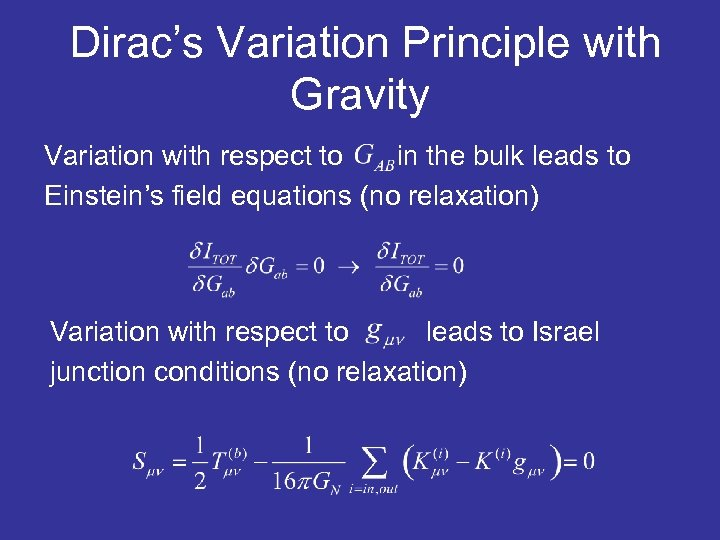Dirac’s Variation Principle with Gravity Variation with respect to in the bulk leads to Einstein’s field equations (no relaxation) Variation with respect to leads to Israel junction conditions (no relaxation)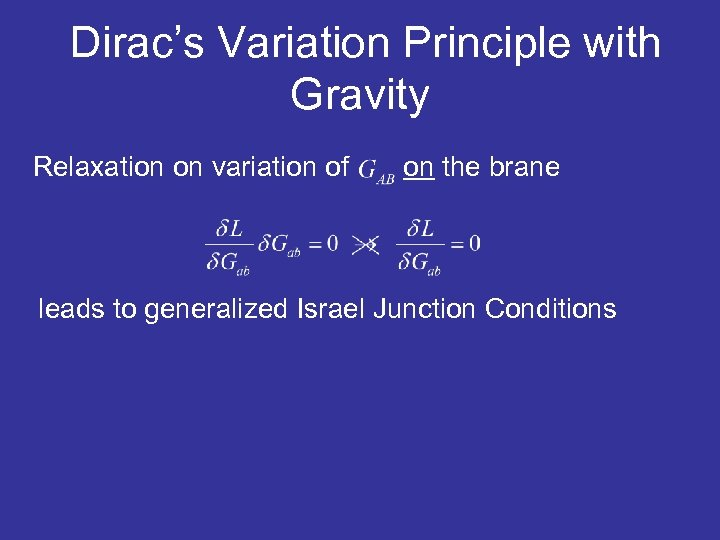Dirac’s Variation Principle with Gravity Relaxation on variation of on the brane leads to generalized Israel Junction ConditionsDirac’s Variation Principle with Gravity Relaxation on variation of on the brane leads to generalized Israel Junction Conditions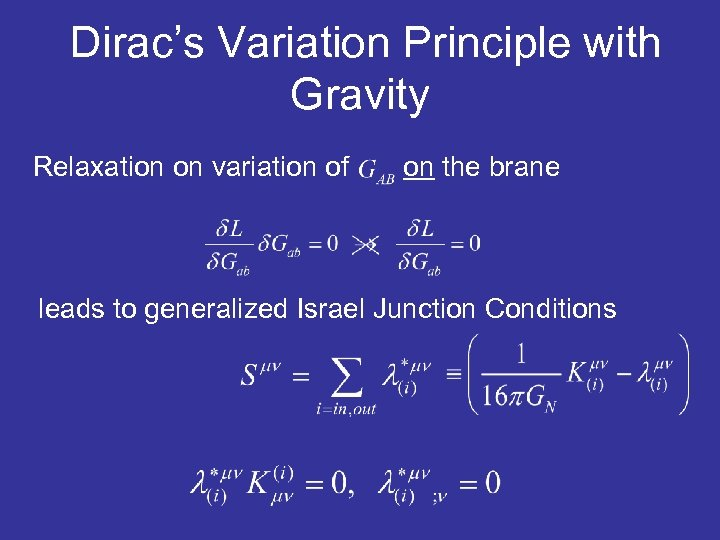Dirac’s Variation Principle with Gravity Relaxation on variation of on the brane leads to generalized Israel Junction Conditions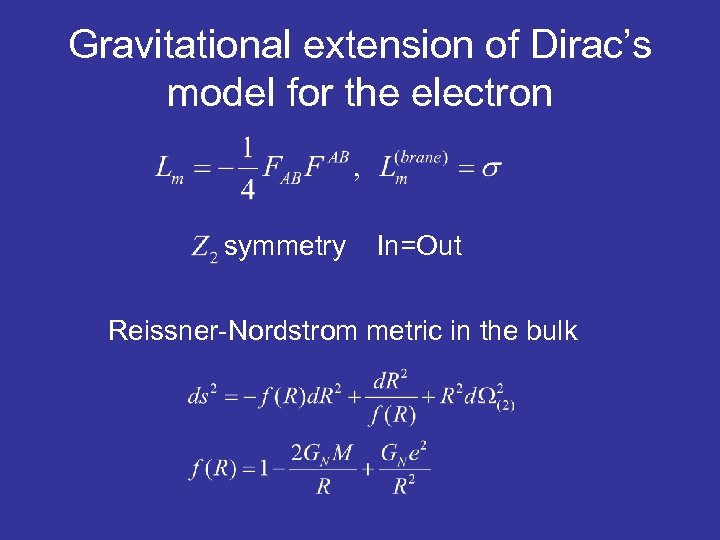Gravitational extension of Dirac’s model for the electron symmetry In=Out Reissner-Nordstrom metric in the bulk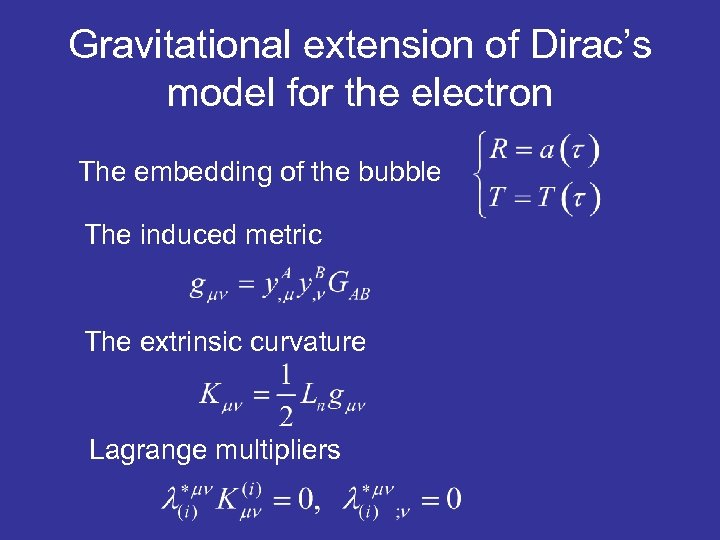Gravitational extension of Dirac’s model for the electron The embedding of the bubble The induced metric The extrinsic curvature Lagrange multipliers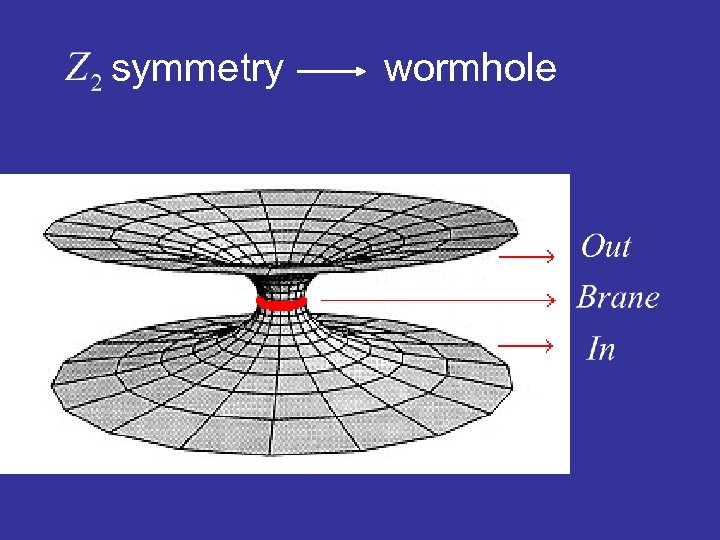symmetry wormhole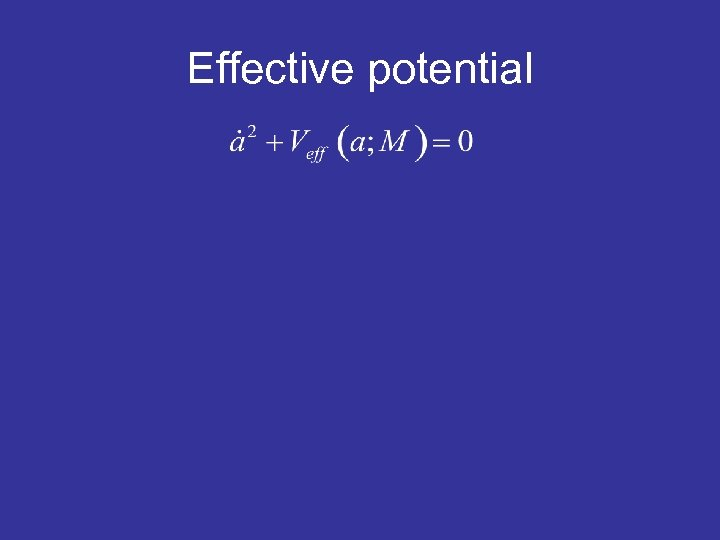Effective potential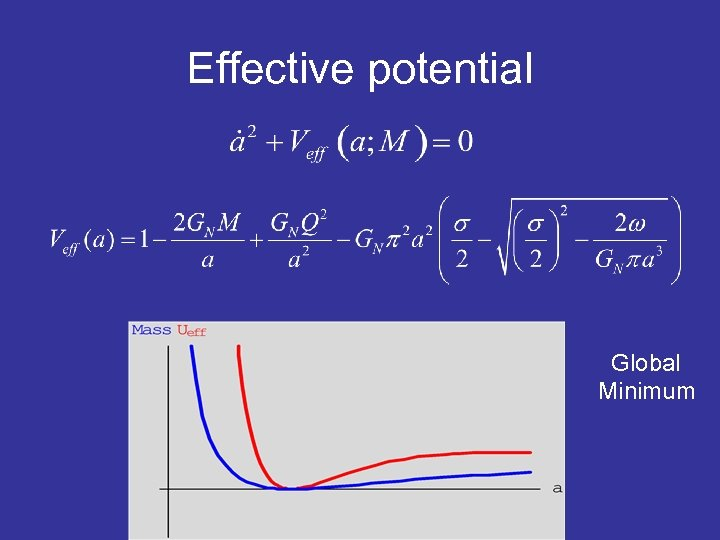Effective potential Global Minimum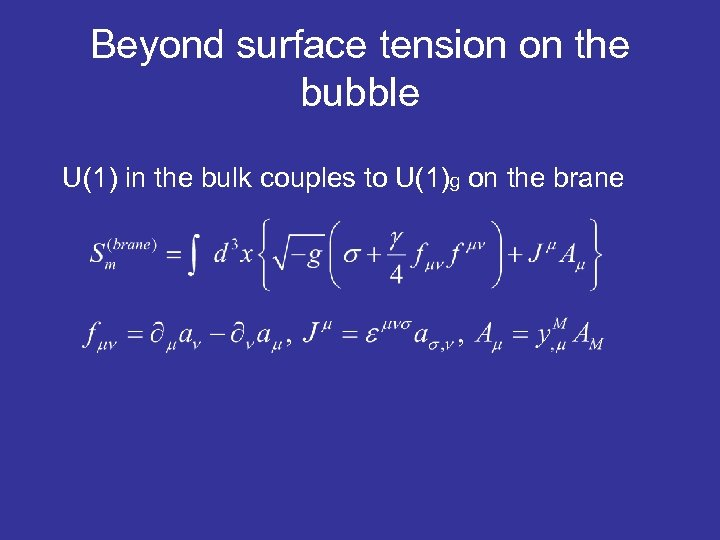Beyond surface tension on the bubble U(1) in the bulk couples to U(1)g on the brane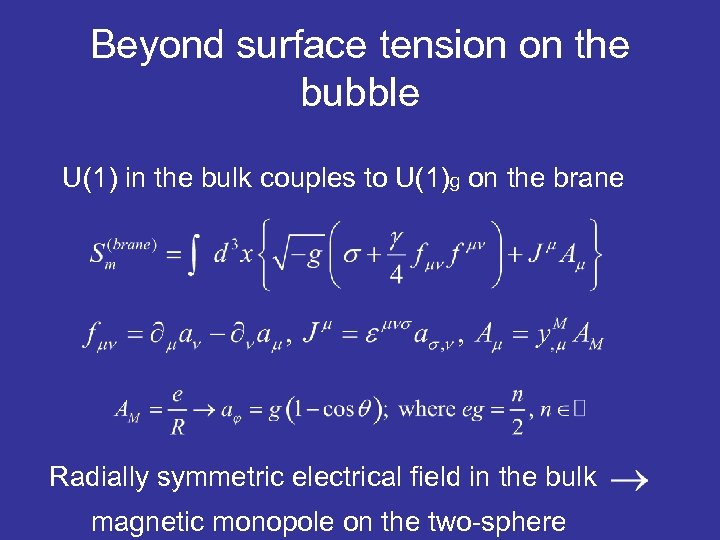Beyond surface tension on the bubble U(1) in the bulk couples to U(1)g on the brane Radially symmetric electrical field in the bulk magnetic monopole on the two-sphere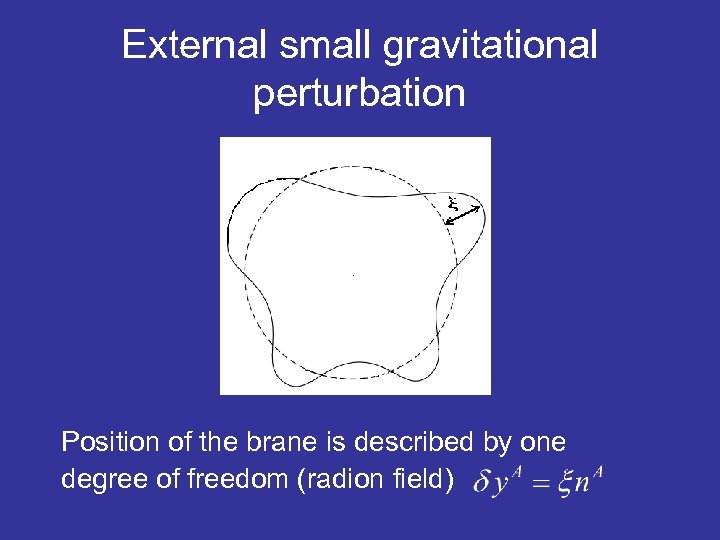External small gravitational perturbation Position of the brane is described by one degree of freedom (radion field)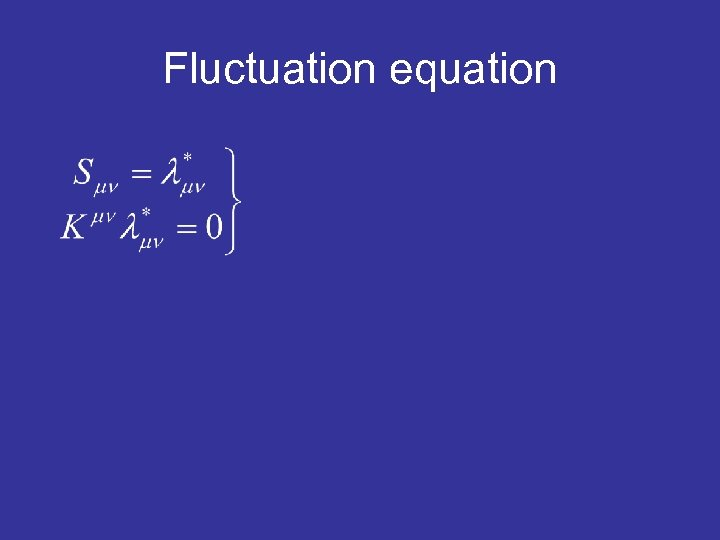Fluctuation equation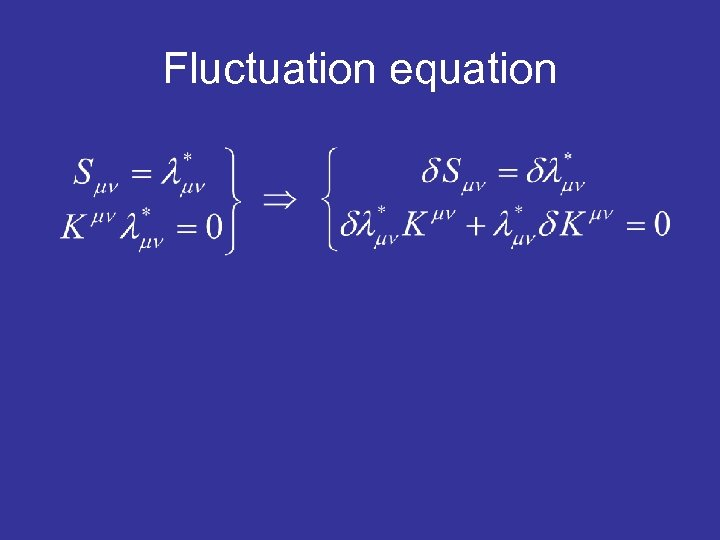Fluctuation equation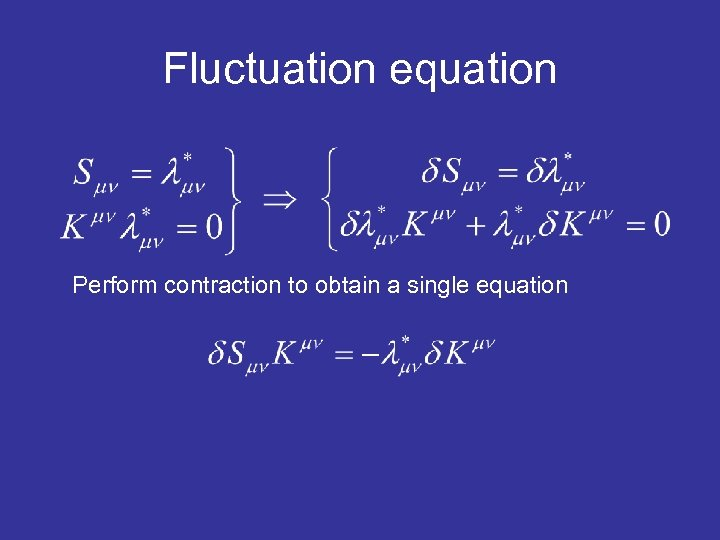Fluctuation equation Perform contraction to obtain a single equation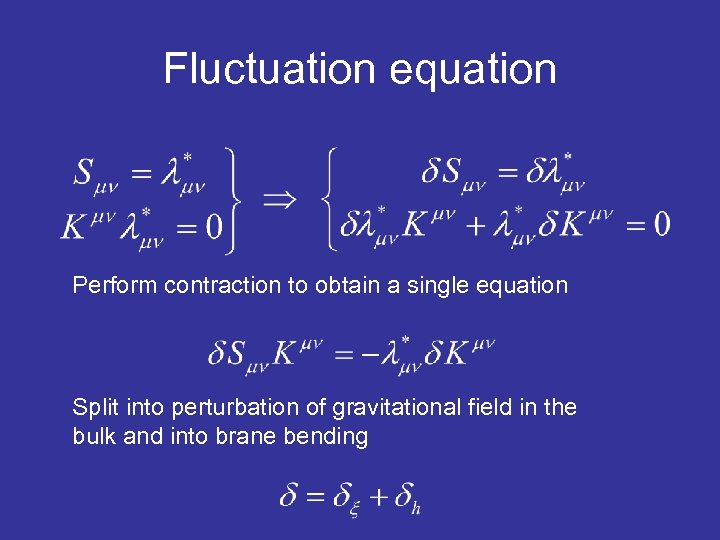Fluctuation equation Perform contraction to obtain a single equation Split into perturbation of gravitational field in the bulk and into brane bending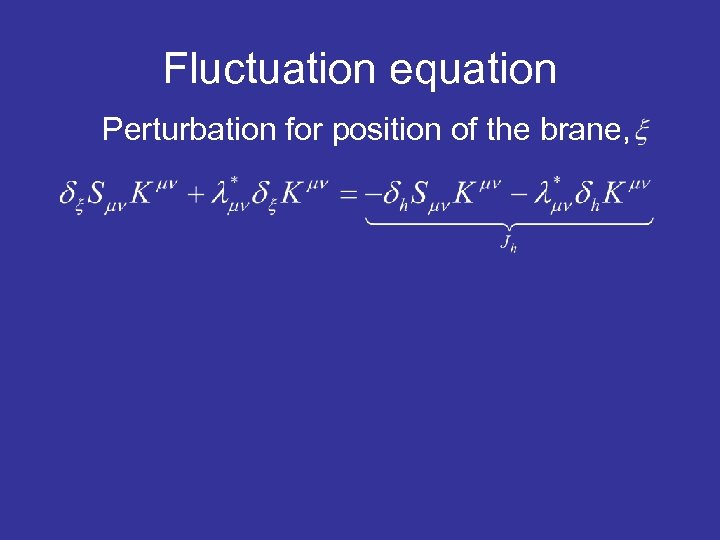Fluctuation equation Perturbation for position of the brane,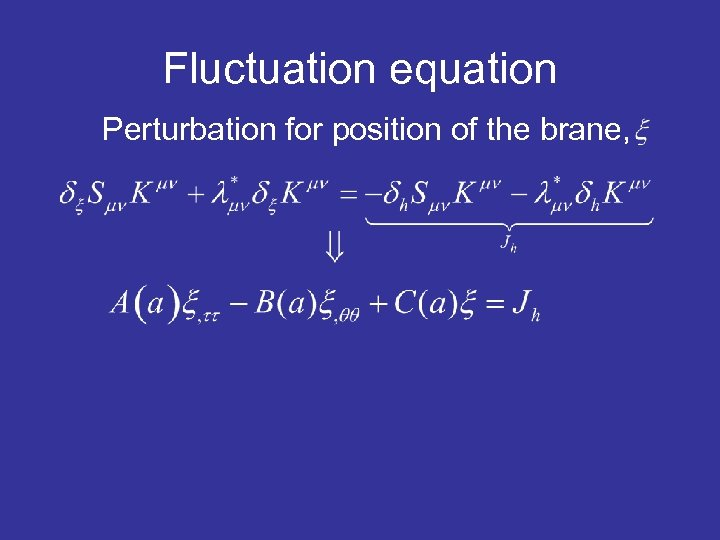Fluctuation equation Perturbation for position of the brane,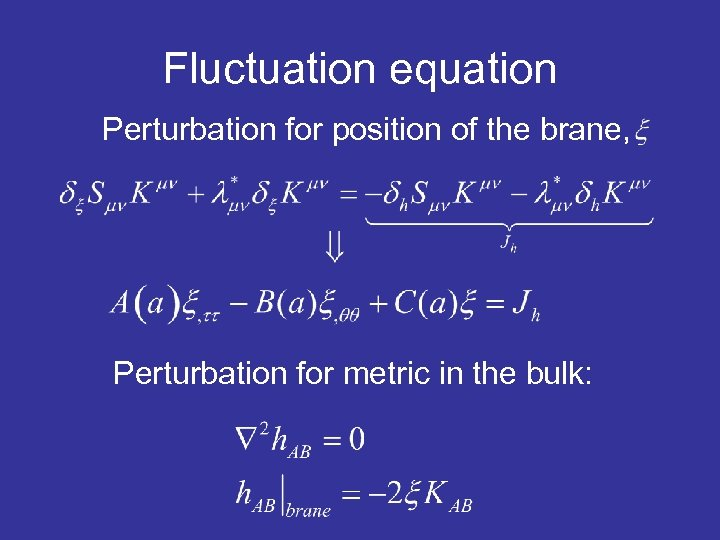Fluctuation equation Perturbation for position of the brane, Perturbation for metric in the bulk: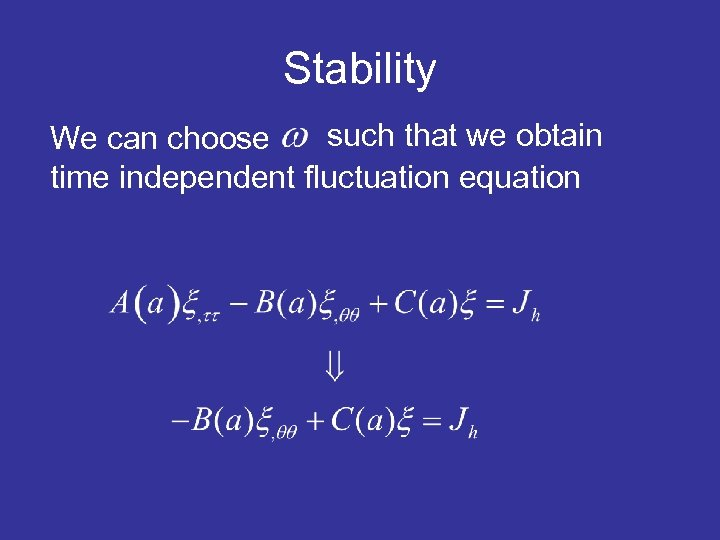Stability such that we obtain We can choose time independent fluctuation equation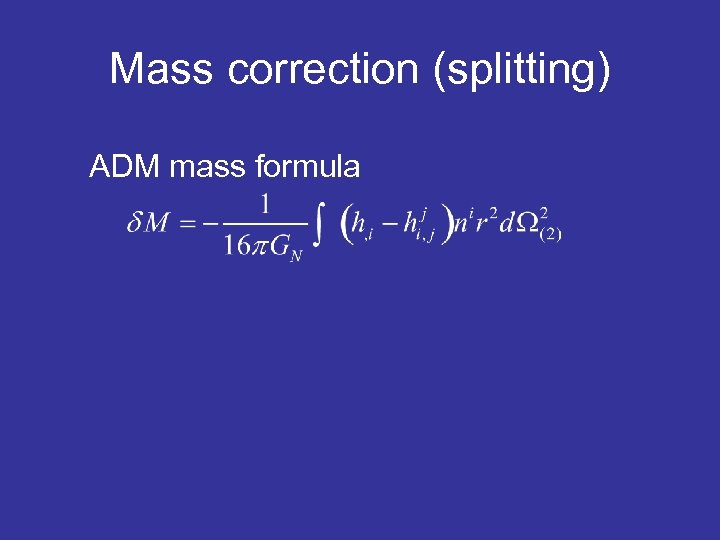Mass correction (splitting) ADM mass formula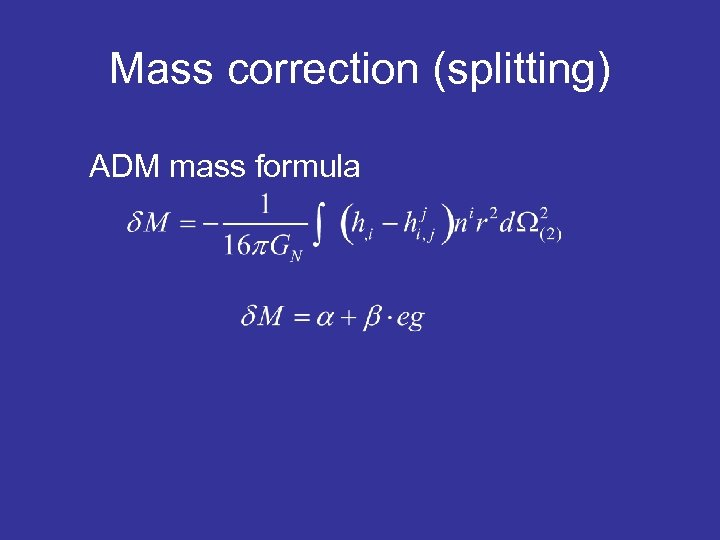Mass correction (splitting) ADM mass formula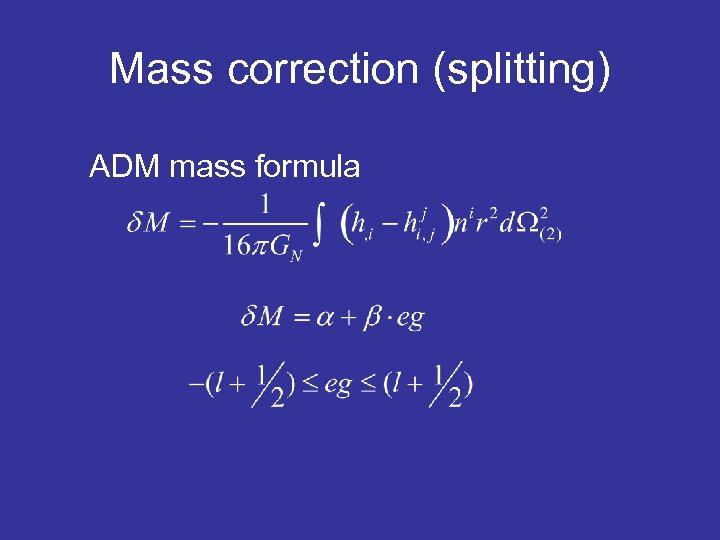Mass correction (splitting) ADM mass formula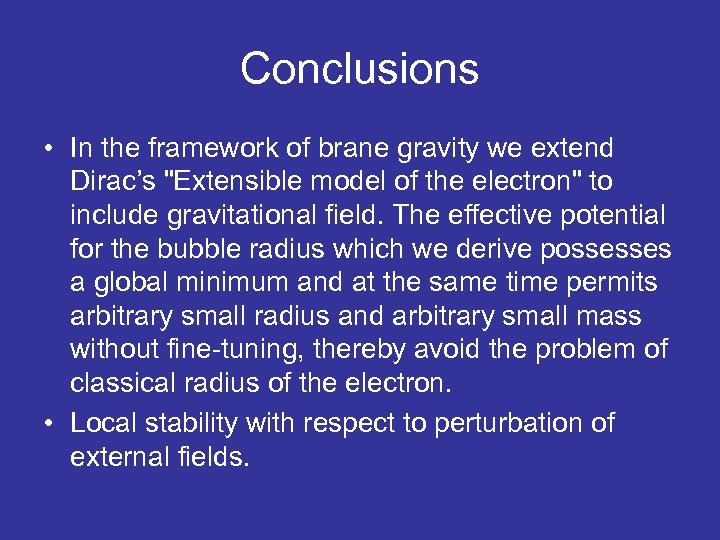Conclusions • In the framework of brane gravity we extend Dirac’s "Extensible model of the electron" to include gravitational field. The effective potential for the bubble radius which we derive possesses a global minimum and at the same time permits arbitrary small radius and arbitrary small mass without fine-tuning, thereby avoid the problem of classical radius of the electron. • Local stability with respect to perturbation of external fields.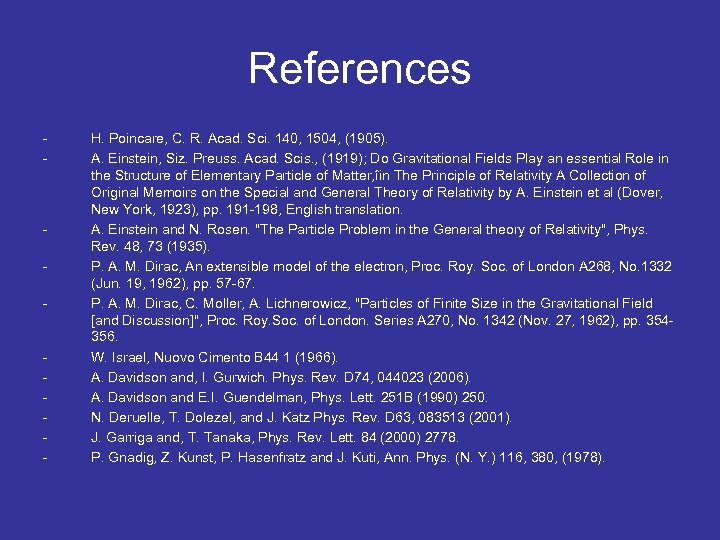References - - - H. Poincare, C. R. Acad. Sci. 140, 1504, (1905). A. Einstein, Siz. Preuss. Acad. Scis. , (1919); Do Gravitational Fields Play an essential Role in the Structure of Elementary Particle of Matter, îin The Principle of Relativity A Collection of Original Memoirs on the Special and General Theory of Relativity by A. Einstein et al (Dover, New York, 1923), pp. 191 -198, English translation. A. Einstein and N. Rosen. "The Particle Problem in the General theory of Relativity", Phys. Rev. 48, 73 (1935). P. A. M. Dirac, An extensible model of the electron, Proc. Roy. Soc. of London A 268, No. 1332 (Jun. 19, 1962), pp. 57 -67. P. A. M. Dirac, C. Moller, A. Lichnerowicz, "Particles of Finite Size in the Gravitational Field [and Discussion]", Proc. Roy. Soc. of London. Series A 270, No. 1342 (Nov. 27, 1962), pp. 354356. W. Israel, Nuovo Cimento B 44 1 (1966). A. Davidson and, I. Gurwich. Phys. Rev. D 74, 044023 (2006). A. Davidson and E. I. Guendelman, Phys. Lett. 251 B (1990) 250. N. Deruelle, T. Dolezel, and J. Katz Phys. Rev. D 63, 083513 (2001). J. Garriga and, T. Tanaka, Phys. Rev. Lett. 84 (2000) 2778. P. Gnadig, Z. Kunst, P. Hasenfratz and J. Kuti, Ann. Phys. (N. Y. ) 116, 380, (1978).